Rotational energy and entropy due to rotational motion.

The entropy due to the rotational motion of the molecules of a gas can be calculated.

Linear molecules: as was pointed out, any rotating molecule has a set of allowed rotational energies. For a linear molecule the allowed rotational energies of a molecule of moment of inertia I are given approximated by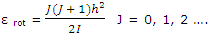Furthermore, the number of states corresponding to a given value of J is given by 2J + 1. These features of the rotational energy patterns allow the rotational partition function to be deduced. This result can be used to obtain the rotational entropy contribution. The rotational contribution to the entropy, which must be added to the rotational contribution, is given by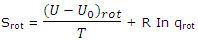The partition function for rotation of a linear molecule obtained is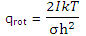For a linear molecule, which has just 2 rotational degrees of freedom, the value of U - U0 for rotation was found, with this expression, to be RT. The rotational entropy of a diatomic or a linear polyatomic molecule can thus be written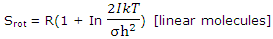When numerical values are inserted for the constants, the rotational contributions of linear molecules to the entropy of ideal gases are given by

rot (J K-1 mol-1) = 877.37 + 8.3144 (In I + In T - In σ) [I in kg m2]

Example: calculate the 25°C rotational entropy of 1 mol of CO molecules. The moment of inertia of a CO molecule, measured by method given is 14.50 × 10-47 kg m2.

Solution: substitution in eq. and recognizing that σ = 1, gives

rot (J K-1 mol-1) = 877.37 + 8.3144[In (14.50 × 10-47) + In 298.15]

= 877.37 + 8.3144 (-105.55 + 5.70)

= 47.17 J K -1 mol-1

For comparison, the translational entropy of 1 mol of CO at 25°C and a pressure of 1 bar is calculated, to be 150.472 J K-1 mol-1.

The much greater translational entropy contribution (compared with the rotational entropy contribution) can be understood in terms of the much closer spacing of the translational energy levels and therefore the much larger number of translational states throughout which the molecules are distributed.

Nonlinear molecules: it is applicable to all diatomic molecules and all linear molecules. Generally shaped molecules, with 3 rather than 2 rotational degrees of freedom, require the use of 3/2 RTfor the rotational energy and the rotational partition function for nonlinear molecules given. For gases composed of such molecules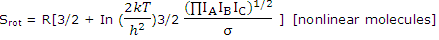With numerical values this becomes

rot (J K-1 mol-1) = 1320.83 + 4.157 In IAIBIC + 12.471 In T - 8.3143 In σ [IA, IB, IC in kg m2]

Limitations: these equations cannot be applied to molecules with very low moments of inertia or at very low temperatures. In both cases the spacing of the energy levels becomes appreciable compared with the thermal energy, and the integration that produced, for example, is not valid.

#### Related Questions in Chemistry

• ##### Q :Problem related to molality Help me to

Help me to solve this problem. What is the molality of a solution which contains 18 g of glucose (C6,H12, O6) in 250 g of water:  (a) 4.0 m (b) 0.4 m (c) 4.2 m (d) 0.8 m

• ##### Q :Ionic radius of chloride ion The edge

The edge length of the unit cell of Nacl crystal lattice is 552 pm. If ionic radius of sodium ion is 95. What is the ionic radius of chloride ion:(a) 190 pm  (b) 368 pm  (c) 181 pm  (d) 276 pm     <

• ##### Q :Chemical formula of detergent Describe

Describe the chemical formula of detergent?

• ##### Q :What is protein in Chemistry Illustrate

Illustrate what is protein in Chemistry?

• ##### Q :Entropy is entropy on moleculare basis

is entropy on moleculare basis relates to the tras.,vib.,and rotational motions?

• ##### Q :F-centres If a electron is present in

If a electron is present in place of anion in a crystal lattice, then it is termed as: (a) Frenkel defect  (b) Schottky defect  (c) Interstitial defects (d) F-centre Answer: (d) When electrons are trapped in anion vacancies, thes

• ##### Q :Question based on strength of solution

Help me to go through this problem. On dissolving 1 mole of each of the following acids in 1 litre water, the acid which does not give a solution of strength 1N is: (a) HCl (b) Perchloric acid (c) HNO3 (d) Phosphoric acid

• ##### Q :Strength of Nacl in solution To 5.85gm

To 5.85gm of Nacl one kg of water is added to prepare of solution. What is the strength of Nacl in this solution (mol. wt. of nacl = 58.5)? (a) 0.1 Normal (b) 0.1 Molal (c) 0.1 Molar (d) 0.1 FormalAnswer:

• ##### Q :Mole fraction of benzene Choose the

Choose the right answer from following. In a solution of 8.7g benzene C6H6 and 46.0 gm toluene ,(C6, H5, CH3) the mole fraction of benzene in this solution is: (a)1/6 (b)1/5 (c)1/2 (d)1/3

• ##### Q :Net charge of a non-ionized atom

Describe the net charge of a non-ionized atom?